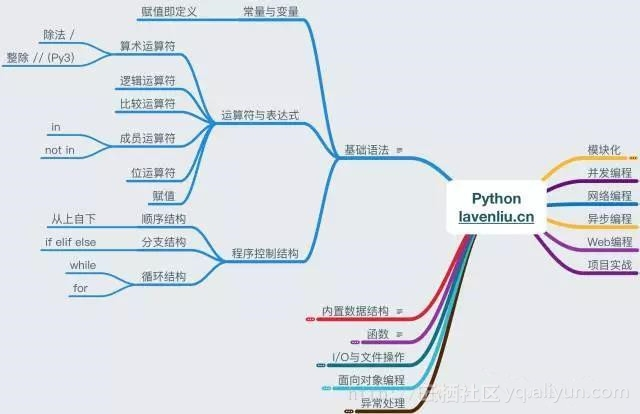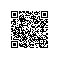# Python基础语法-常量与变量Python是一门强类型的动态语言。

a = 3 # 给一个对象3赋予变量a

+ 变量 是Python解释器系统表中的一些条目, 它们是用来指向对象的。
+ 对象 是一些列的被分配的内存, 有足够的空间存储与之相关的数据类型。
+ 引用 自动地跟随在变量与对象之间。

a = 3 # a是一个整型数据
a = ‘spam’ # 现在是一个字符串了
a = 1.23 # 现在是一个浮点数了

a = 3
b = a

a = 3
b = a
a = ‘spam’

L1 = [2, 3, 4]
L2 = L1

L1是一个列表且包含2、3、4三个对象。列表中的元素通过它们的位置进行访问，所以L1指向对象2，它是列表L1的第一个对象。当然列表也是一个对象，就像其他的整数与字符串。当进行上面的两个赋值后，L1与L2都指向了同一个共享的对象。

L1 = 24

L1 = [2, 3, 4] # 一个可变的对象
L2 = L1 # L2与L1指向了同样的对象
L1 = 24 # 一个原地改变的操作
L1 # L1已经改变了
[24, 3, 4]
L2 # L2也是与L1相同
[24, 3, 4]

d1 = {‘a’: 1, ‘b’: 2}
d2 = d1
d1
{‘b’: 2, ‘a’: 1}
d2
{‘b’: 2, ‘a’: 1}
d1[‘a’] = ‘a’
d2
{‘b’: 2, ‘a’: ‘a’}

L1 = [2, 3, 4]
L2 = L1[:] # 制作L1的拷贝
L1 = 24
L1
[24, 3, 4]
L2 # L2并没有改变
[2, 3, 4]

L = [1, 2, 3]
M = L # M与L指向相同的对象
L == M # 具有相同的值
True
L is M # 依然相同
True
id(L)
4338913608
id(M)
4338913608

L = [1, 2, 3]
M = [1, 2, 3] # M与L指向不同的对象（赋值意味着产生新的对象）
L == M # 具有相同的值
True
L is M # 但是是不同的对象
False
id(L)
4338913480
id(M)
4338915208

X = 42
Y = 42 # X与Y应该是两个不同的对象
id(X)
4297547872
id(Y)
4297547872
X == Y
True
X is Y # but anyhow: 是缓存在作祟！
True

X = 1234567
Y = 1234567
X == Y
True
X is Y
False
id(X)
4336930640
id(Y)
4338529328使用钉钉扫一扫加入圈子
+ 订阅